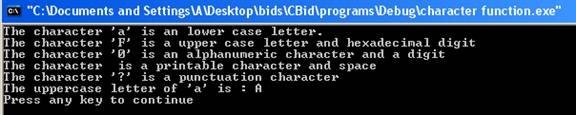C++ Character Functions

The C++ char functions are extremely useful for testing and transforming characters. These functions are widely used and accepted. In order to use character functions header file <cctype> is included into the program. Some of the commonly used character functions are:

• isalnum()-  The function isalnum()  returns nonzero value if its argument is either an alphabet or integer. If the character is not an integer or alphabet then it returns zero.

•  isalpha() - The function isalpha() returns nonzero if the character is an uppercase or lower case letter otherwise it returns zero.

•  iscntrl() - The function iscntrl() returns nonzero if the character is a control character otherwise it returns zero.

•  isdigit()- The function isdigit() returns nonzero if the character is a digit, i.e. through 0 to 9. It returns zero for non digit character.

• isgraph()- The function isgraph() returns nonzero if the character is any printable character other than space otherwise it returns zero.

• islower()- The function islower() returns nonzero for a lowercase letter otherwise it returns zero.

• isprint()- The function isprint() returns nonzero for printable character including space otherwise it returns zero.

• isspace()- The function isspace() returns nonzero for space, horizontal tab, newline character, vertical tab, formfeed, carriage return; otherwise it returns zero.

• ispunct()- The function ispunct() returns nonzero for punctuation characters otherwise it returns zero. The punctuation character excludes alphabets, digits and space.

• isupper() - The function isupper() returns nonzero for an uppercase letter otherwise it returns zero.

• tolower()- The function tolower() changes the upper case letter to its equivalent lower case letter. The character other than upper case letter remains unchanged.

• toupper()- The function toupper() changes the lower case letter to its equivalent upper case letter. The character other than lower case letter remains unchanged.

• isxdigit() - The function isxdigit() returns nonzero for hexadecimal digit i.e. digit from 0 to 9, alphabet 'a' to 'f' or 'A' to 'F' otherwise it returns zero.

Here is a program which illustrates the working of character functions.

#include<iostream>

#include<cctype>

using namespace std;

int main ()

{

char ch='a';

char ch1='Z';

char ch2=' ';

char ch3='0';

char ch4='?';

char ch5='F';

if(isalpha(ch))

{

if(islower(ch))

{

cout << "The character 'a' is an lower case letter." << endl;

}

}

if(isupper(ch5))

{

if(isxdigit(ch5))

{

cout << "The character 'F' is a upper case letter and hexadecimal digit" << endl;

}

}

if(isalnum(ch3))

{

if(isdigit(ch3))

{

cout << "The character '0' is an alphanumeric character and a digit" << endl;

}

}

if(isprint(ch2))

{

if(isspace(ch2))

{

if(isgraph(ch2))

{

cout << "The character is a graphic character" << endl;

}

else

{

cout << "The character " " is a printable character and space" << endl;

}

}

}

if(ispunct(ch4))

{

cout << "The character '?' is a punctuation character" << endl;

}

cout << "The uppercase letter of 'a' is : " << static_cast<char>(toupper(ch)) << endl;

return(0);

}

The result of the program is:-The statement

#include<cctype>

I

includes a header file <cctype> into the program. The statement

if(isalpha(ch))

checks whether character ch is alphanumeric. It returns nonzero value as 'a' is alphanumeric. The statement

if(islower(ch))

checks whether character ch is a lower case letter. It returns nonzero value as 'a' is lower case letter. The statement

if(isupper(ch5))

checks whether character ch5 is a upper case letter. It returns nonzero value as 'F' is a upper case letter. The statement

if(isxdigit(ch5))

checks whether character ch5 is a hexadecimal digit. It returns nonzero value as 'F' is a hexadecimal digit. The statement

if(isalnum(ch3))

checks whether character ch3 is a alphanumeric character. It returns nonzero value as '0' is an alphanumeric character. The statement

if(isdigit(ch3))

checks whether character ch3 is a digit. It returns nonzero value as '0' is digit. The statement

if(isprint(ch2))

checks whether character ch2 is a printable character . It returns nonzero value as character ch2 ' '  is a printable character. The statement

if(isspace(ch2))

checks whether character ch2 is a space character . It returns nonzero value as character ch2 ' '  is space. The statement

if(isgraph(ch2))

checks whether character ch2 is a graphical character . It returns zero value as character ch2 is not a graphical character. The statement

if(ispunct(ch4))

checks whether character ch4 is punctuation character. It returns nonzero value as character ch4 '?' is a question mark. The statement

cout << "The uppercase letter of 'a' is : " << static_cast<char>(toupper(ch)) << endl;

displays the upper case equivalent of letter 'a'. The function toupper(ch) converts lower case letter to upper case letter and it returns integer value therefore type casting is used to convert integer type value to character type value. If type casting is not used then it will return the ASCII code of upper case letter.

Go to the previous lesson or proceed to the next lesson